# 4. Visualization of nearest neighbor distance distributions

## Introduction

This tutorial shows how euclidean nearest neighbor distances in the geographic space or feature space can be calculated and visualized using CAST. This type of visualization allows to assess whether training data feature a representative coverage of the prediction area and if cross-validation (CV) folds (or independent test data) are adequately chosen to be representative for the prediction locations.

See e.g. Meyer and Pebesma (2022) and Mila et al. (2022) for further discussion on this topic.

## Sample data

As example data, we use two different sets of global virtual reference data: One is a spatial random sample and in the second example, reference data are clustered in geographic space (see Meyer and Pebesma (2022) for more discussions on this).

library(CAST)
library(caret)
library(raster)
library(sf)
library(rnaturalearth)
library(ggplot2)

### Prediction area

The prediction area is the entire global land area, i.e. we could imagine a prediction task where we aim at making global predictions based on the set of reference data.

ee <- st_crs("+proj=eqearth")
co <- ne_countries(returnclass = "sf")
co.ee <- st_transform(co, ee)

### Spatial random sample

Then, we simulate the random sample and visualize the data on the entire global prediction area.

sf_use_s2(FALSE)
set.seed(10)
pts_random <- st_sample(co, 1000)
### See points on the map:
ggplot() + geom_sf(data = co.ee, fill="#00BFC4",col="#00BFC4") +
geom_sf(data = pts_random, color = "#F8766D",size=0.5, shape=3) +
guides(fill = FALSE, col = FALSE) +
labs(x = NULL, y = NULL)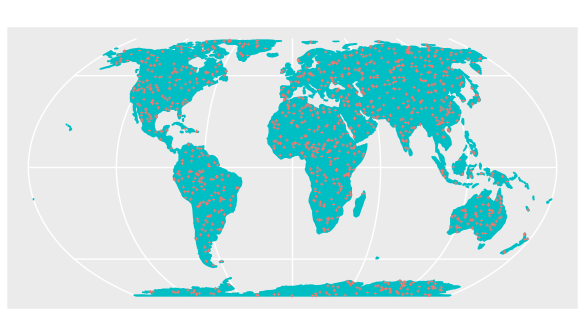### Clustered sample

As second data set we use a clustered design of the same size.

set.seed(10)
sf_use_s2(FALSE)
pts_clustered <- clustered_sample(co, 1000, 20, 8)

ggplot() + geom_sf(data = co.ee, fill="#00BFC4",col="#00BFC4") +
geom_sf(data = pts_clustered, color = "#F8766D",size=0.5, shape=3) +
guides(fill = FALSE, col = FALSE) +
labs(x = NULL, y = NULL)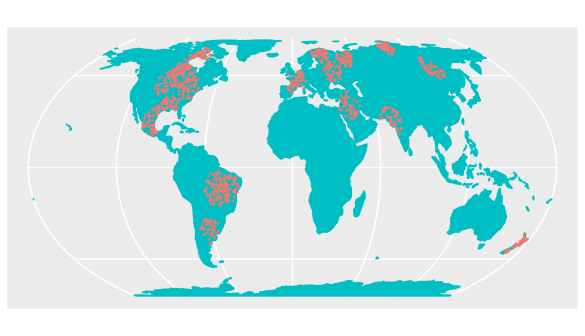## Distances in geographic space

Then we can plot the distributions of the spatial distances of reference data to their nearest neighbor (“sample-to-sample”) with the distribution of distances from all points of the global land surface to the nearest reference data point (“sample-to-prediction”). Note that samples of prediction locations are used to calculate the sample-to-prediction nearest neighbor distances. Since we’re using a global case study here, throughout this tutorial we use sampling=Fibonacci to draw prediction locations with constant point density on the sphere.

dist_random <- plot_geodist(pts_random,co,
sampling="Fibonacci",
showPlot = FALSE)
dist_clstr <- plot_geodist(pts_clustered,co,
sampling="Fibonacci",
showPlot = FALSE)

dist_random$plot+scale_x_log10(labels=round)+ggtitle("Randomly distributed reference data")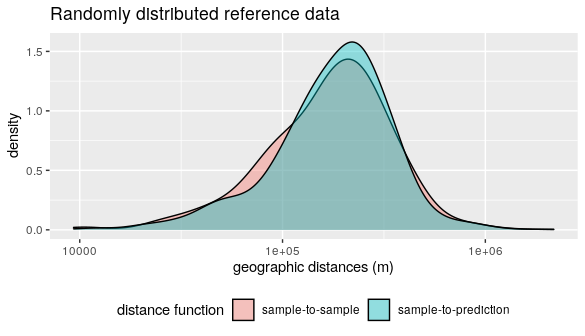dist_clstr$plot+scale_x_log10(labels=round)+ggtitle("Clustered reference data")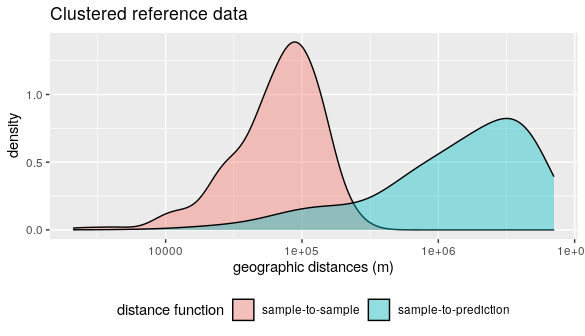Note that for the random data set the nearest neighbor distance distribution of the training data is quasi identical to the nearest neighbor distance distribution of the prediction area. In comparison, the second data set has the same number of training data but these are heavily clustered in geographic space. We therefore see that the nearest neighbor distances within the reference data is rather small. Prediction locations, however, are on average much further away.

### Accounting for cross-validation folds

#### Random Cross-validation

Let’s use the clustered data set to show how the distribution of spatial nearest neighbor distances during cross-validation can be visualized as well. Therefore, we first use the “default” way of a random 10-fold cross validation where we randomly split the reference data into training and test (see Meyer et al., 2018 and 2019 to see why this might not be a good idea).

randomfolds <- caret::createFolds(1:nrow(pts_clustered))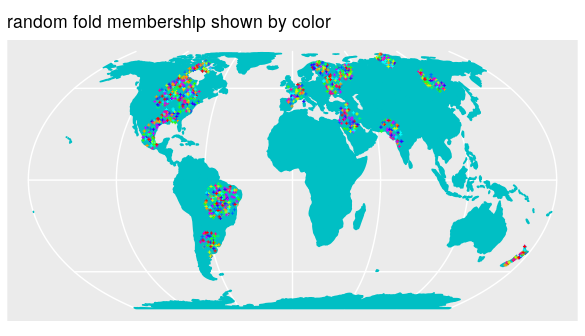dist_clstr <- plot_geodist(pts_clustered,co,
sampling="Fibonacci",
cvfolds= randomfolds,
showPlot=FALSE)
dist_clstr$plot+scale_x_log10(labels=round)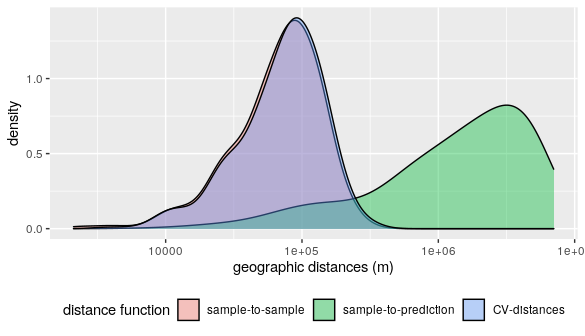Obviously the CV folds are not representative for the prediction locations (at least not in terms of distance to a nearest training data point). I.e. when these folds are used for performance assessment of a model, we can expect overly optimistic estimates because we only validate predictions in close proximity to the reference data. #### Spatial Cross-validation This, however, should not be the case but the CV performance should be regarded as representative for the prediction task. Therefore, we use a spatial CV instead. Here, we use a leave-cluster-out CV, which means that in each iteration, one of the spatial clusters is held back. spatialfolds <- CreateSpacetimeFolds(pts_clustered,spacevar="parent",k=length(unique(pts_clustered$parent)))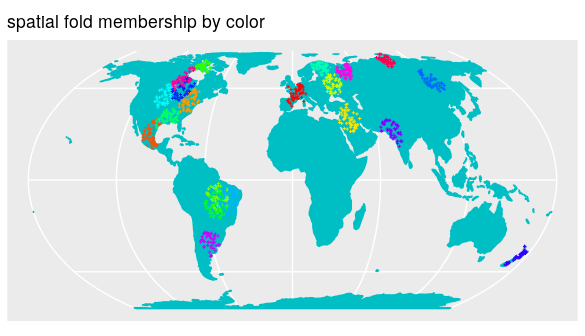dist_clstr <- plot_geodist(pts_clustered,co,
sampling="Fibonacci",
cvfolds= spatialfolds$indexOut, showPlot=FALSE) dist_clstr$plot+scale_x_log10(labels=round)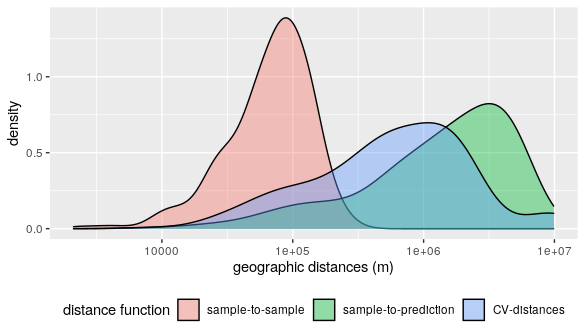See that this fits the nearest neighbor distribution of the prediction area much better (See Mila et al. (2022) for a better idea to do the spatial CV). Note that plot_geodist also allows inspecting independent test data instead of cross validation folds. See ?plot_geodist.

## Distances in feature space

So far we compared nearest neighbor distances in geographic space. We can also do so in feature space. Therefore, a set of bioclimatic variables are used (https://www.worldclim.org) as features (i.e. predictors) in this virtual prediction task.

predictors_global <- stack(system.file("extdata","bioclim_global.grd",package="CAST"))

plot(predictors_global)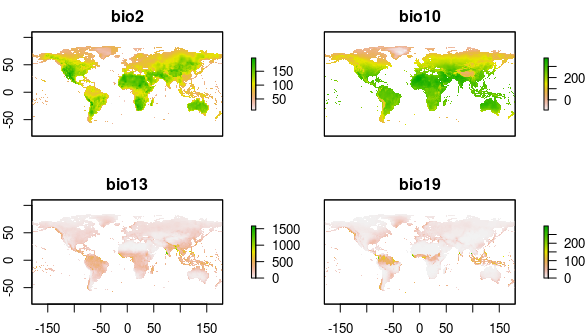Then we visualize nearest neighbor feature space distances under consideration of cross-validation.

# use random CV:
dist_clstr_rCV <- plot_geodist(pts_clustered,predictors_global,
type = "feature",
sampling="Fibonacci",
cvfolds = randomfolds,
showPlot=FALSE)

# use spatial CV:
dist_clstr_sCV <- plot_geodist(pts_clustered,predictors_global,
type = "feature", sampling="Fibonacci",
cvfolds = spatialfolds$indexOut, showPlot=FALSE) # Plot results: dist_clstr_rCV$plot+scale_x_log10(labels=round)+ggtitle("Clustered reference data and random CV")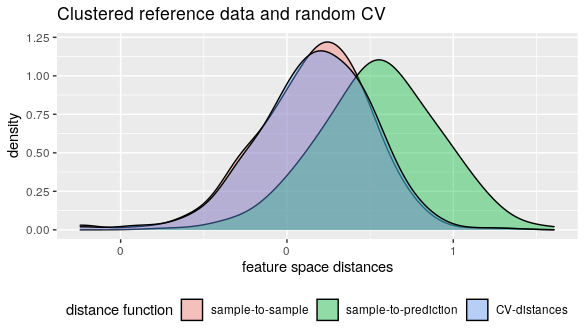dist_clstr_sCV\$plot+scale_x_log10(labels=round)+ggtitle("Clustered reference data and spatial CV")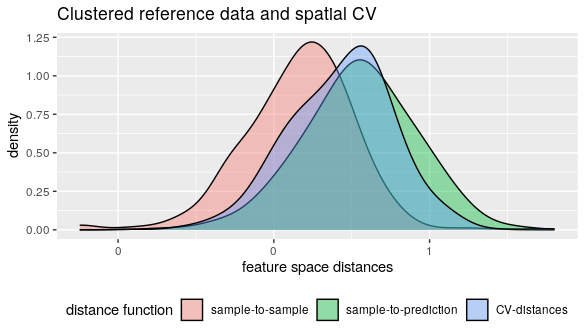With regard to the chosen predictor variables we see that again the nearest neighbor distance of the clustered training data is rather small, compared to what is required during prediction. Again the random CV is not representative for the prediction locations while the spatial CV is doing a better job.